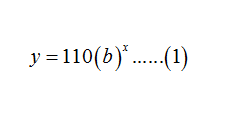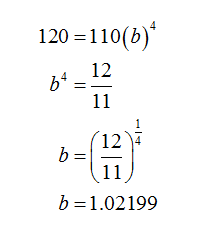# A pair of​ women's running shoes cost \$110 in 2005 and the same shoes cost​ \$120 in 2009 due to inflation. a. Develop an exponential model to describe the rate of inflation over this time period.b. If inflation continues at the same​ rate, use your model in part​ (a) to estimate the price of the shoes in 2015. a. The rate of inflation can be modeled by the following exponential​ equation, where the model solves for the inflated price y after time x. y=110(__)x​(Round to four decimal places as​ needed.) b. The estimated price of the running shoes in 2015 will be \$__.​(Round to two decimal places as​ needed.)

Question
4 views
A pair of​ women's running shoes cost \$110 in 2005 and the same shoes cost​ \$120 in 2009 due to inflation.

a. Develop an exponential model to describe the rate of inflation over this time period.
b. If inflation continues at the same​ rate, use your model in part​ (a) to estimate the price of the shoes in 2015.

a. The rate of inflation can be modeled by the following exponential​ equation, where the model solves for the inflated price y after time x.

y=110(__)x
​(Round to four decimal places as​ needed.)

b. The estimated price of the running shoes in 2015 will be \$__.
​(Round to two decimal places as​ needed.)
check_circle

Step 1

It is given that the pair of woman running shoes costs \$110 in 2005 and the same shoes cost \$120 in 2009 due to inflation.

And, the given exponential model isStep 2

To find:

• The exponential model over the time period 2005 to 2009.
• Using, model in part (a) estimate the price of shoes in 2015, if the inflation continuous at the same rate.
Step 3
• Since at x=0 in 2005, the cost of shoes is \$110 and the same shoes cost \$120 in 2009 at x=4.

Therefore, from (1), satisfying x=4 and plugging y=120....

### Want to see the full answer?

See Solution

#### Want to see this answer and more?

Solutions are written by subject experts who are available 24/7. Questions are typically answered within 1 hour.*

See Solution
*Response times may vary by subject and question.
Tagged in

### Algebra